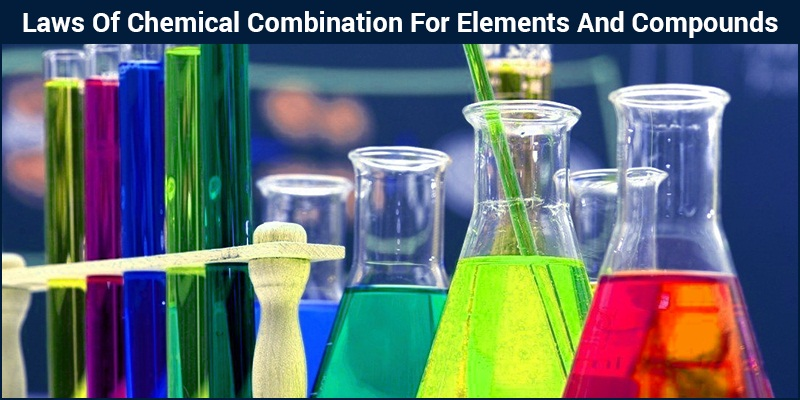# Laws Of Chemical Combination For Elements And Compounds

Chemistry is the study of the transformation of matter from one form to the other. These transformations often occur as a result of the combination of two different types of matter. The combination of different elements to form compounds is governed by certain basic rules. These rules are referred to as laws of chemical combination. There are five basic laws of chemical combination that govern the chemical combinations of elements:1. Law of Conservation of Mass

In simple terms, this law states that matter can neither be created nor destroyed. In other words, the total mass, that is, the sum of mass of reacting mixture and the products formed remains  constant. Antoine Lavoisier gave this law in the year 1789 based on the data he obtained after carefully studying numerous combustion reactions.

2. Law of Definite Proportions

Joseph Proust, a French chemist stated that the proportion of elements by weight in a given compound will always remain exactly the same. In simple terms we can say that, irrespective of its source, origin or its quantity, the percent composition of elements by weight in a given compound will always remain the same.

3. Law of Multiple Proportions

This law states that if two elements combine to form more than one compound, the masses of these elements in the reaction are in the ratio of small whole numbers. This law was given by Dalton in the year 1803.

4.  Gay Lussac’s Law of Gaseous Volumes

In 1808, Gay Lussac gave this law based on his observations. This law states that when gases are produced or combine in a chemical reaction, they do so in simple ratio by volume given that all the gases are at same temperature and pressure. This law can be considered as another form of law of definite proportions. The only difference between these two laws of chemical combination is that Gay Lussac’s Law is stated with respect to volume while law of definite proportions is stated with respect to mass.